# Maximum-likelihood method

(diff) ← Older revision | Latest revision (diff) | Newer revision → (diff)

One of the fundamental general methods for constructing estimators of unknown parameters in statistical estimation theory.

Suppose one has, for an observationwith distributiondepending on an unknown parameter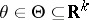, the task to estimate. Assuming that all measures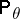are absolutely continuous relative to a common measure, the likelihood function is defined by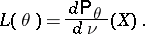The maximum-likelihood method recommends taking as an estimator forthe statistic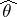defined by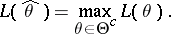is called the maximum-likelihood estimator. In a broad class of cases the maximum-likelihood estimator is the solution of a likelihood equation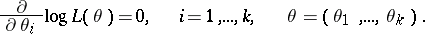(1)

Example 1. Let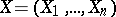be a sequence of independent random variables (observations) with common distribution,. If there is a density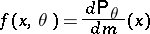relative to some measure, then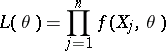and the equations (1) take the form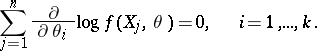(2)

Example 2. In Example 1, letbe the normal distribution with density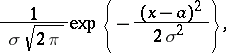where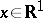,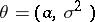,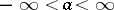,. Equations (2) become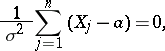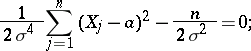and the maximum-likelihood estimator is given by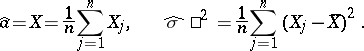Example 3. In Example 1, let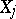take the valuesandwith probabilities,, respectively. Then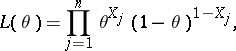and the maximum-likelihood estimator is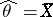.

Example 4. Let the observation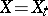be a diffusion process with stochastic differential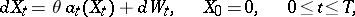whereis a Wiener process andis an unknown one-dimensional parameter. Here (see ),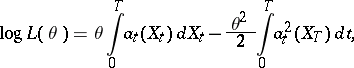There are no definitive reasons for optimality of the maximum-likelihood method and the widespread belief in its efficiency is partially based on the great success with which it has been applied to numerous concrete problems, and partially on rigorously established asymptotic optimality properties. For example, in Example 1, under broad assumptions,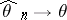with-probability. If the Fisher information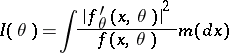exists, then the difference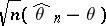is asymptotically normal with parameters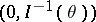, and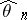, in a well-defined sense, has an asymptotically-minimal mean-square deviation from(see , ).

How to Cite This Entry:
Maximum-likelihood method. Encyclopedia of Mathematics. URL: http://encyclopediaofmath.org/index.php?title=Maximum-likelihood_method&oldid=13827
This article was adapted from an original article by I.A. Ibragimov (originator), which appeared in Encyclopedia of Mathematics - ISBN 1402006098. See original article